﻿ 基于改进隔离罩涡流损耗的磁耦合传动装置温度场分析
 舰船科学技术2017, Vol. 39Issue (12): 36-39PDF

Temperature field analysis of magnetic coupled transmission based on improved eddy current loss of separator
FU Pan, ZHANG Qiang, LIU Xu-hao
Kunming Branch of the 705 Research Institute of CSIC, Kunming 650118, China
Abstract: The magnetic coupling transmission is an effective method for the sealing of the underwater deep tail shaft. When the temperature rise of the titanium alloy isolation cover is exorbitant, it will lead to the deformation of the structure and the demagnetization of the permanent magnet. Based on the working characteristics of the magnetic coupling transmission, the 2D and 3D loss models are established in this paper. We calculated the eddy current loss of the shield under different permanent magnet arrangement to select the minimum loss one. The finite element analysis model of three-dimensional temperature field is established by using Fluent software. We also analyze the transient temperature field of main components such as magnetic coupling drive, isolation cover and permanent magnet when eddy current loss is minimum. The simulation results show that the eddy current loss of the shield reaches the smallest when the permanent magnet is divided into four segments, and the transient temperature of each component satisfies the safety performance requirement.
Key words: magnetic coupling     isolation cover     eddy current loss     transient temperature field
0 引　言

1 几何模型建立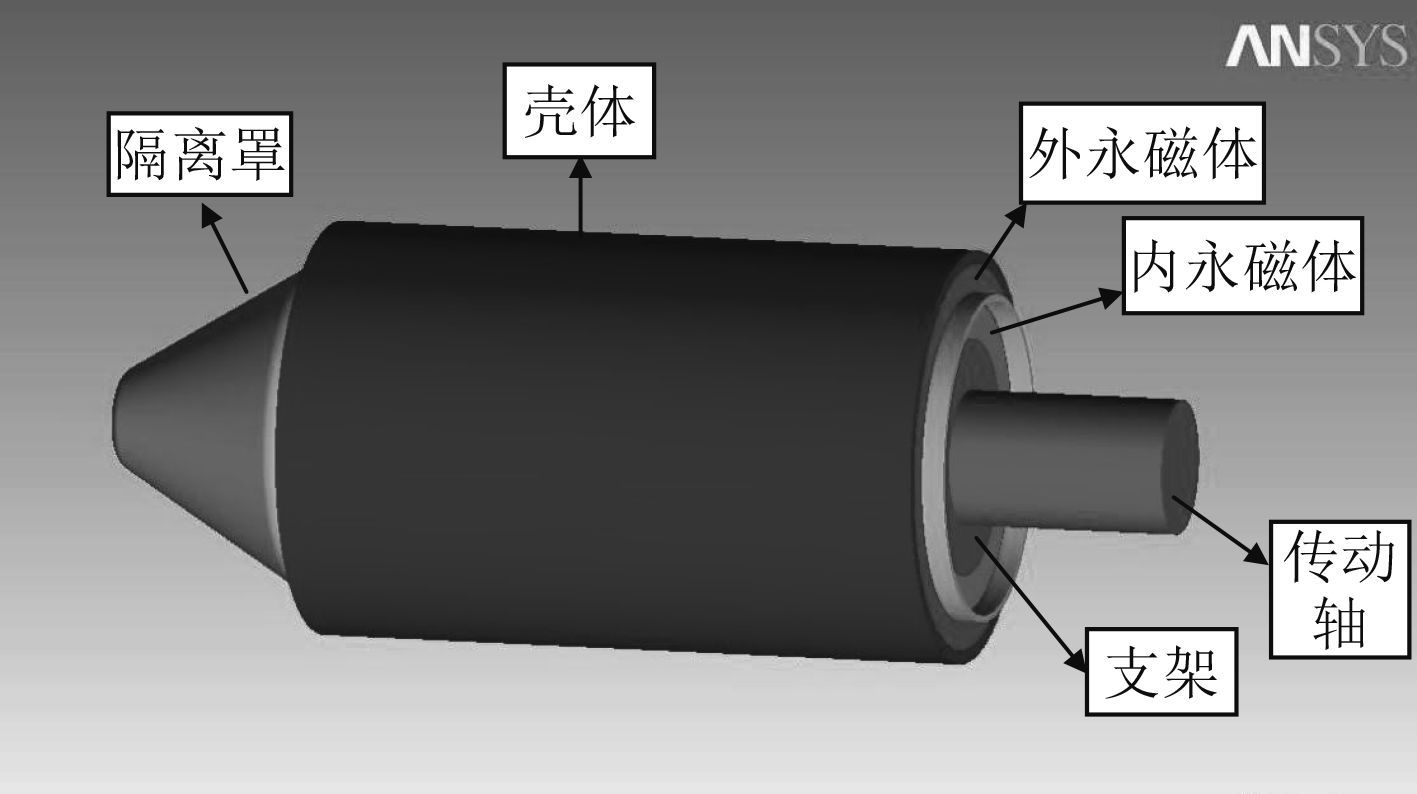图 1 磁耦合传动装置三维几何模型 Fig. 1 The 3D geometric model of magnetic coupling driving device表 1 磁耦合传动装置各部件材料 Tab.1 Each component material of magnetic coupling driving device
2 损耗计算

2.1 隔离罩涡流损耗理论

 ${{{B}}_{\rm{\gamma }}} = {{{B}}_{\rm{\delta }}} \times \sin {\rm{\gamma }}\text{。}$ (1)

 $\frac{{\partial {{{B}}_{\rm{\gamma }}}}}{{\partial {\rm{\beta }}}} = - \frac{1}{{{R}}} \times \frac{{\partial {{E}}}}{{\partial {\rm{\gamma }}}}\text{。}$ (2)

 $\frac{{{{\rm d}E}}}{{{\rm{d\gamma }}}} = - {{R}} \times \frac{{2{\rm{\pi f}}}}{{{P}}}{{{B}}_{\rm{\delta }}} \times {\rm{cos\gamma }}\text{。}$ (3)

 $\int_0^{{{E}}(\gamma )} {dE = - R\frac{{2\pi f}}{P}{B_\sigma }} \int_0^\gamma {\cos } \gamma \times d\gamma \text{，}$ (4)
 ${{E}}\left( {\rm{\gamma }} \right) = - \frac{{2{{R\pi f}}}}{{{P}}}{{{B}}_{\rm{\delta }}}{\rm{sin\gamma }}\text{。}$ (5)

 ${{J}} = - \frac{{2{{\pi Rf\sigma }}}}{{{P}}}{{{B}}_{\rm{\delta }}}{\rm{sin\gamma }}\text{。}$ (6)

 $\begin{split}{{{P}}_{{z}}} =& \int_0^L \int_0^{2 \pi} {\left[{{JR{\rm d}}\left( {{\rm{\beta \gamma }}} \right)} \right]^2}\frac{1}{{\rm{\sigma }}} \times \frac{{{{{\rm d}L}}}}{{{Rd}\left( {{\rm{\beta \gamma }}} \right)}} =\\&4{L}{{R}^3}{{\sigma \beta B}}_{\rm{\delta }}^2\frac{{{{\pi }^3}{{{f}}^3}}}{{{{P}^2}}}\text{。}\end{split}$ (7)

2.2 隔离罩2D和3D损耗模型有限元计算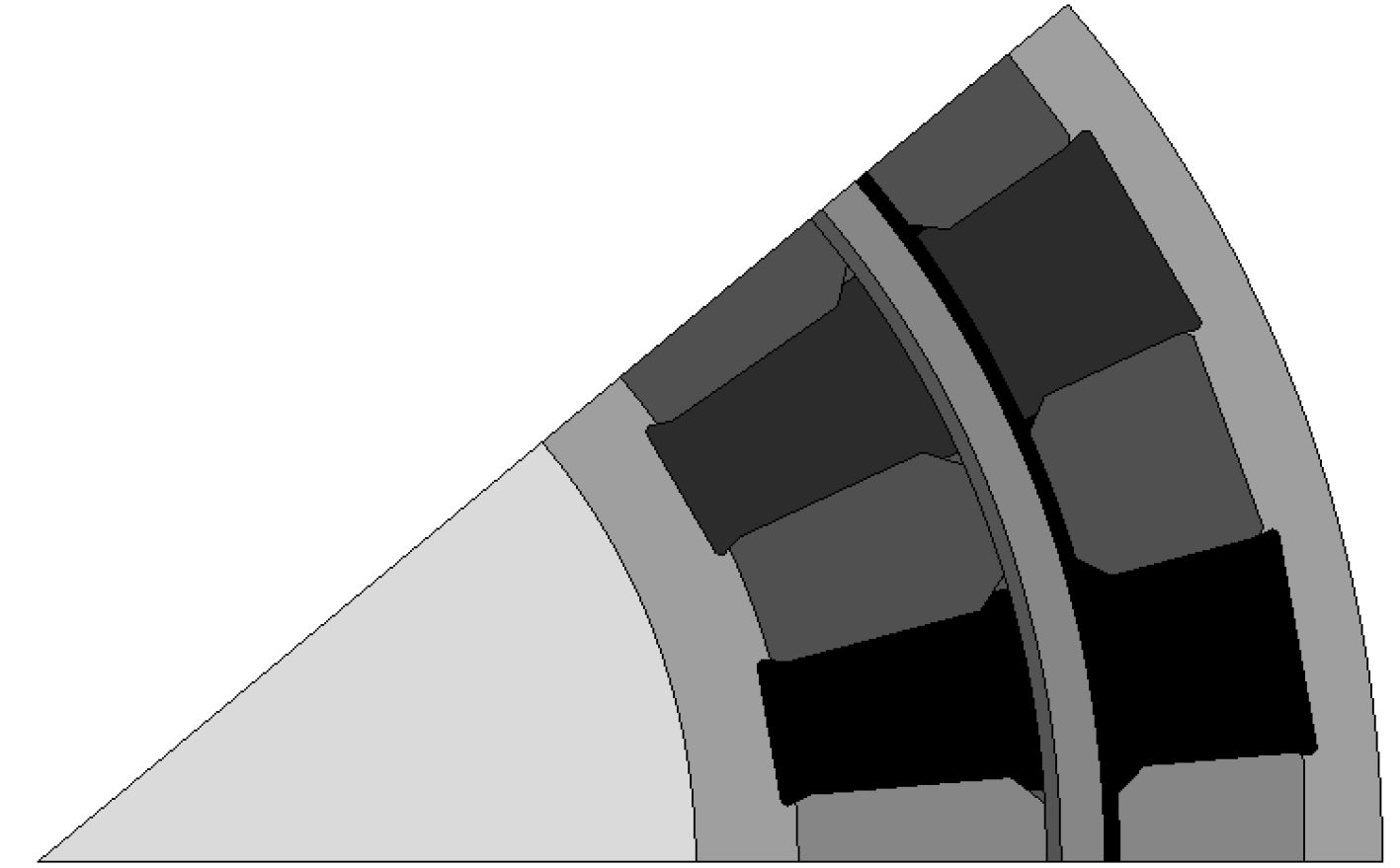图 2 2D整体永磁体损耗模型 Fig. 2 The loss model of 2D permanent magnets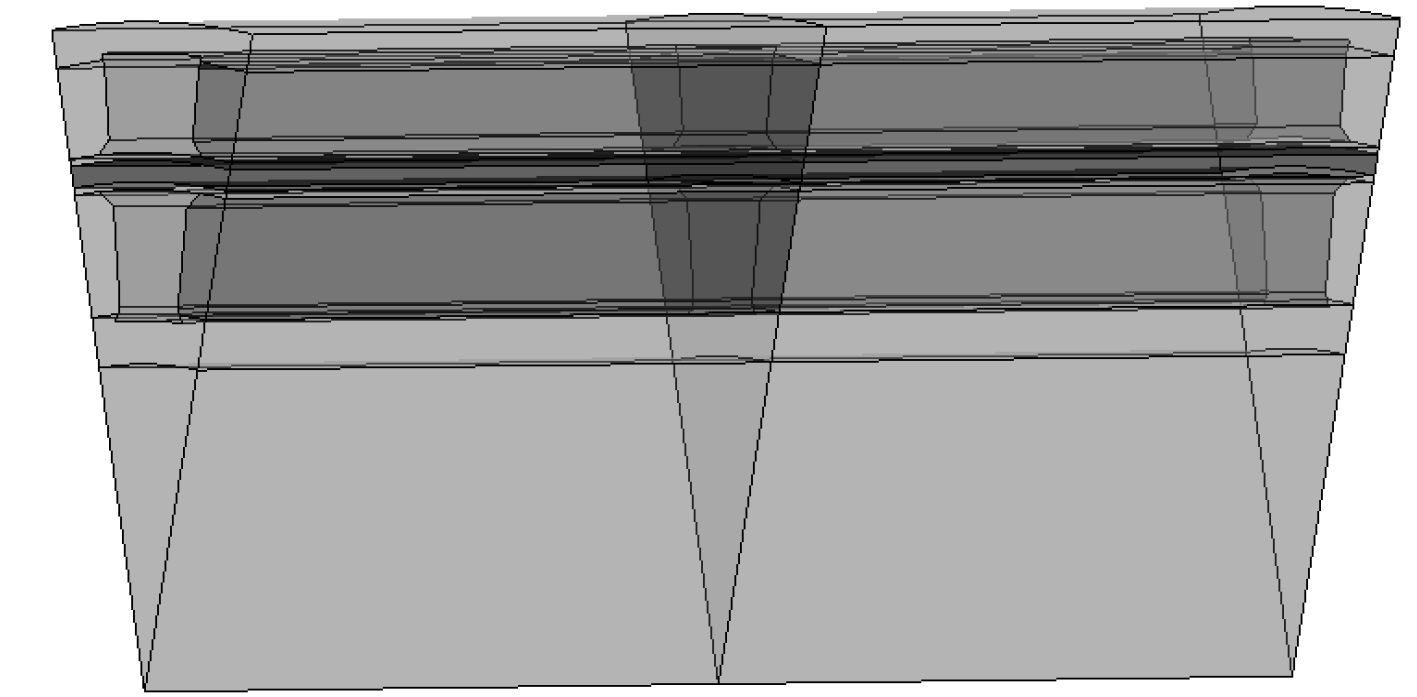图 3 永磁体轴向2段3D模型 Fig. 3 The 3D model of axial two-segment permanent magnets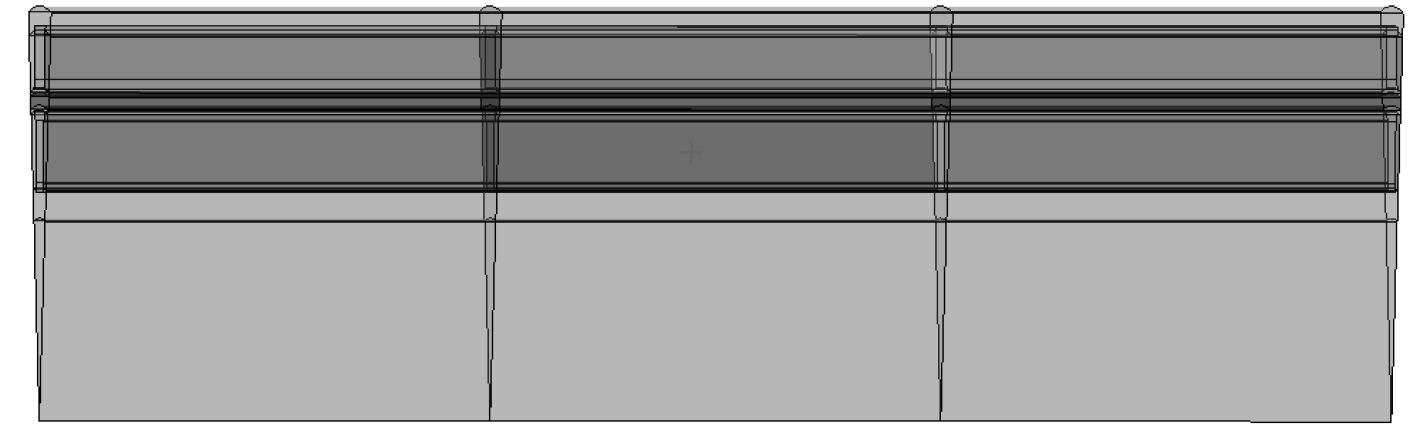图 4 永磁体轴向3段3D模型 Fig. 4 The 3D model of axial three-segment permanent magnets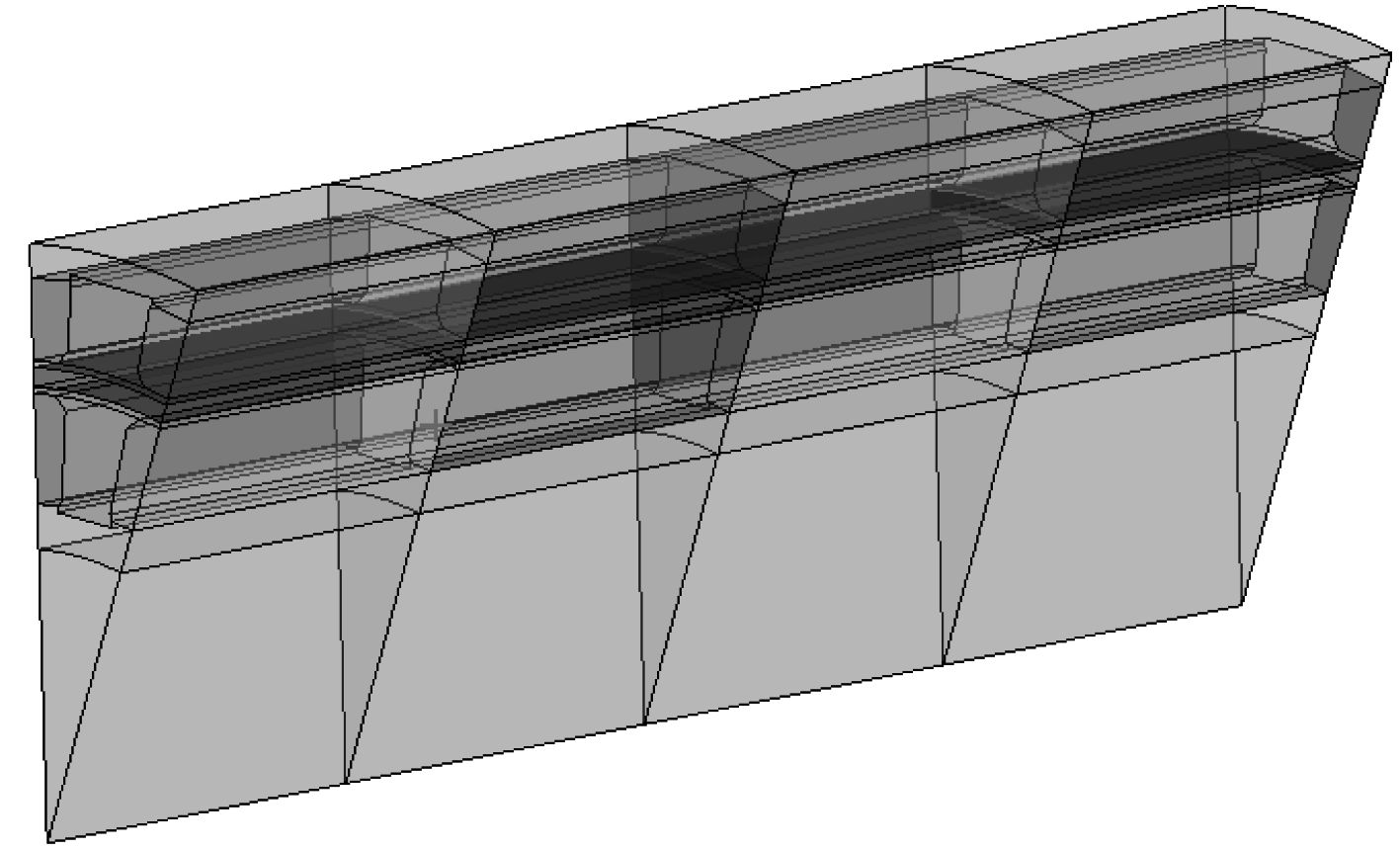图 5 永磁体轴向4段3D模型 Fig. 5 The 3D model of axial four-segment permanent magnets表 2 磁耦合装置2D，3D不同模型下隔离罩涡流损耗 Tab.2 The isolation cover eddy current loss of the 2D, 3D of different model of magnetic coupling device

3 温度场求解

3.1 传热学理论

 $\frac{\partial }{{\partial {{x}}}}\left( {{{k}}\frac{{\partial {{T}}}}{{\partial {{x}}}}} \right) + \frac{\partial }{{\partial {{y}}}}\left( {{{k}}\frac{{\partial {{T}}}}{{\partial {{y}}}}} \right) + {{\dot q}} = {{\rho }}{{{c}}_{{p}}}\frac{{\partial {{T}}}}{{\partial {{t}}}}\text{。}$ (8)

 $\frac{\partial }{{\partial {{x}}}}\left( {{{k}}\frac{{\partial {{T}}}}{{\partial {{x}}}}} \right) + \frac{\partial }{{\partial {{y}}}}\left( {{{k}}\frac{{\partial {{T}}}}{{\partial {{y}}}}} \right) + \frac{\partial }{{\partial {{z}}}}\left( {{{k}}\frac{{\partial {{T}}}}{{\partial {{z}}}}} \right) + {{\dot q}} = {{\rho }}{{{c}}_{{p}}}\frac{{\partial {{T}}}}{{\partial {{t}}}}$ (9)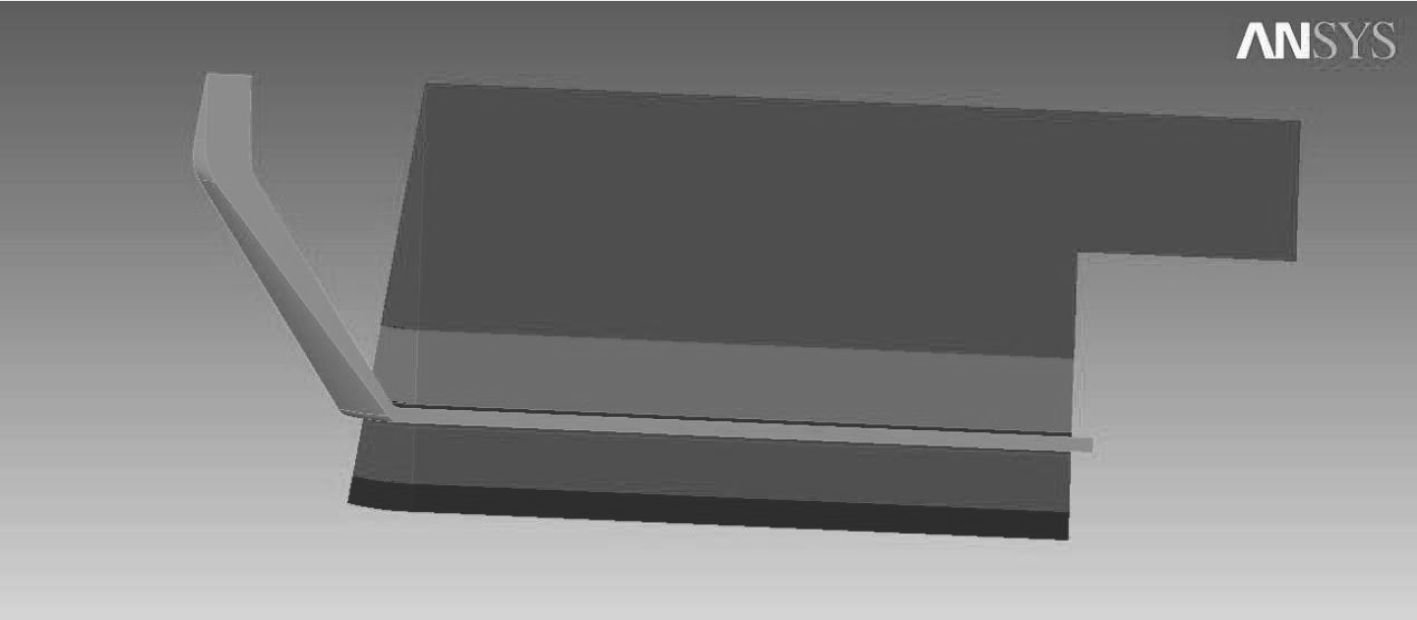图 6 温度场求解1/10模型 Fig. 6 The 1/10 model of temperature field solution
3.2 瞬态温度场求解

 ${{\dot q}} = \frac{{P_Z}}{V}\text{。}$ (10)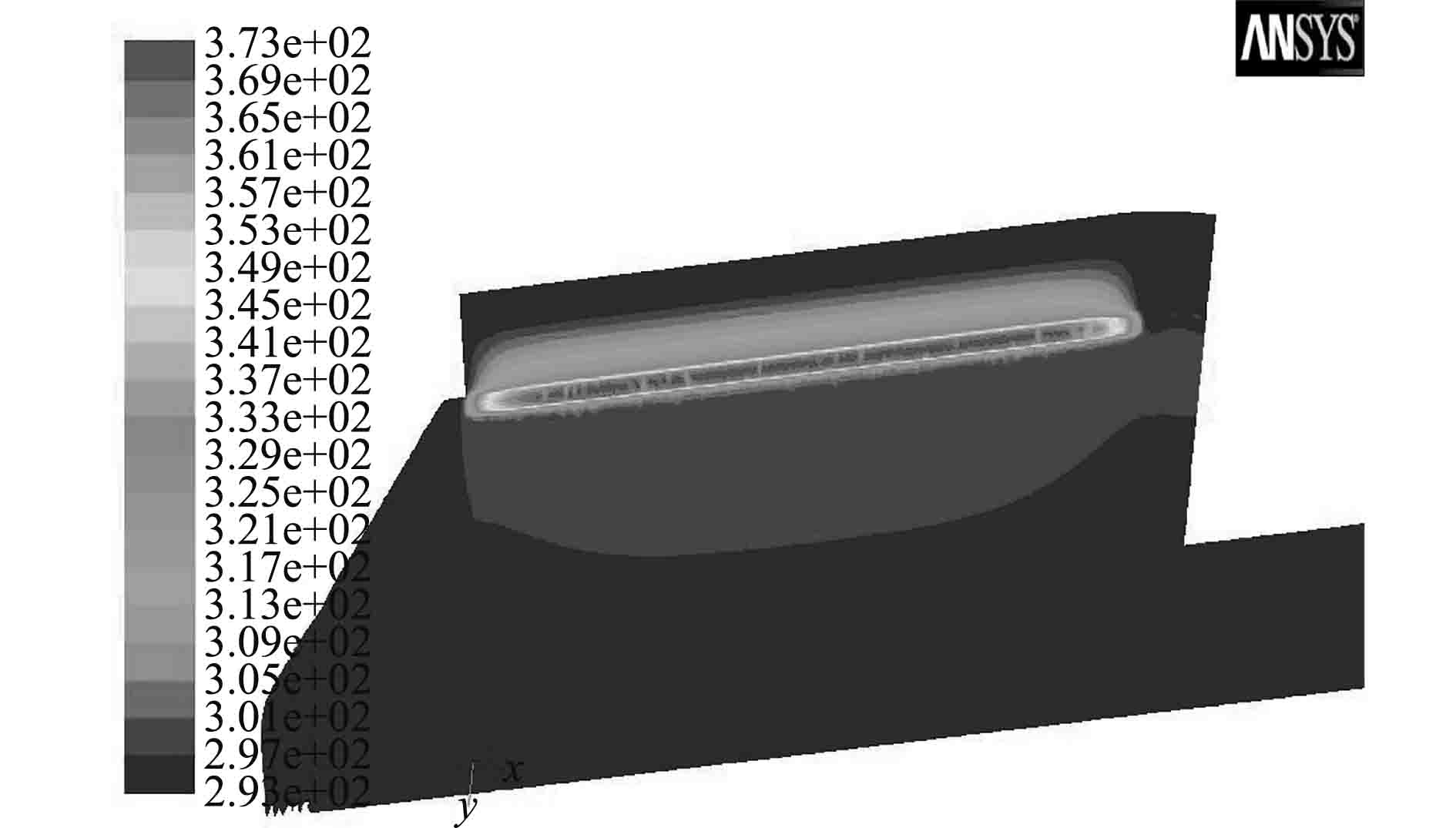图 7 磁耦合传动装置10 min温度场 Fig. 7 The 10 minutes temperature field of magnetic coupling driving device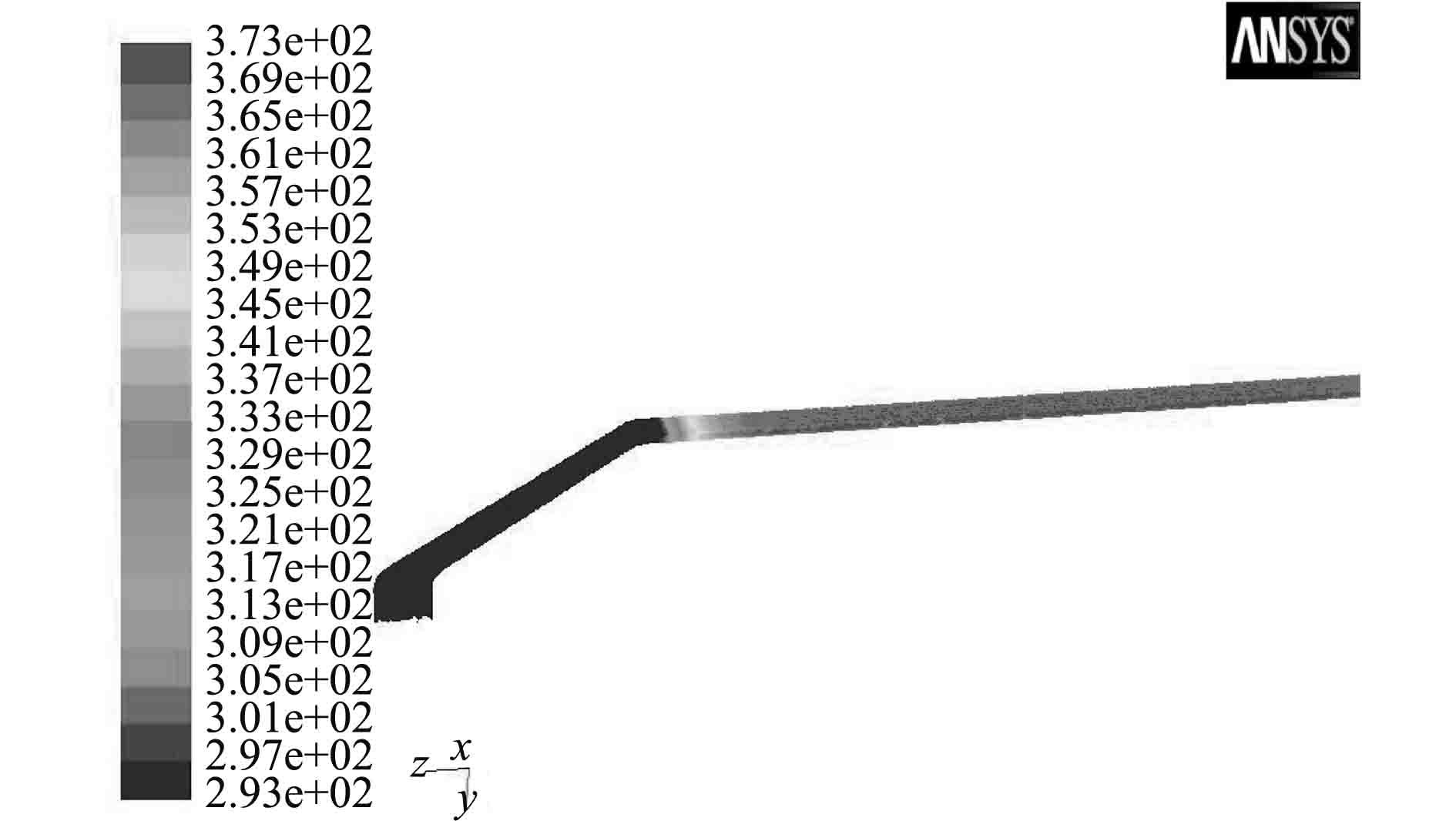图 8 隔离罩10 min温度场 Fig. 8 The 10 minutes temperature field of isolation cover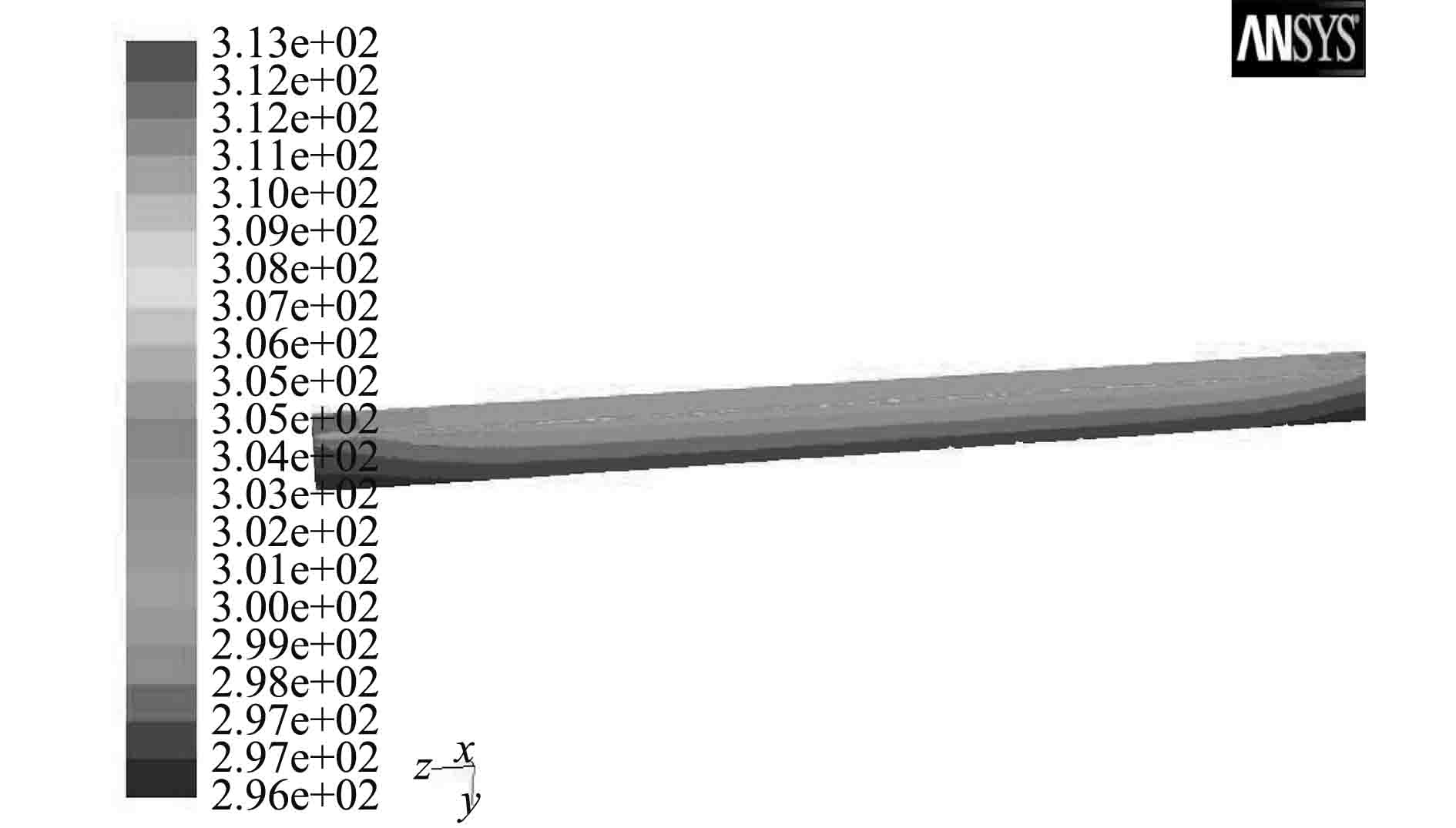图 9 内永磁体10 min温度场 Fig. 9 The 10 minutes temperature field of internal peranment magnet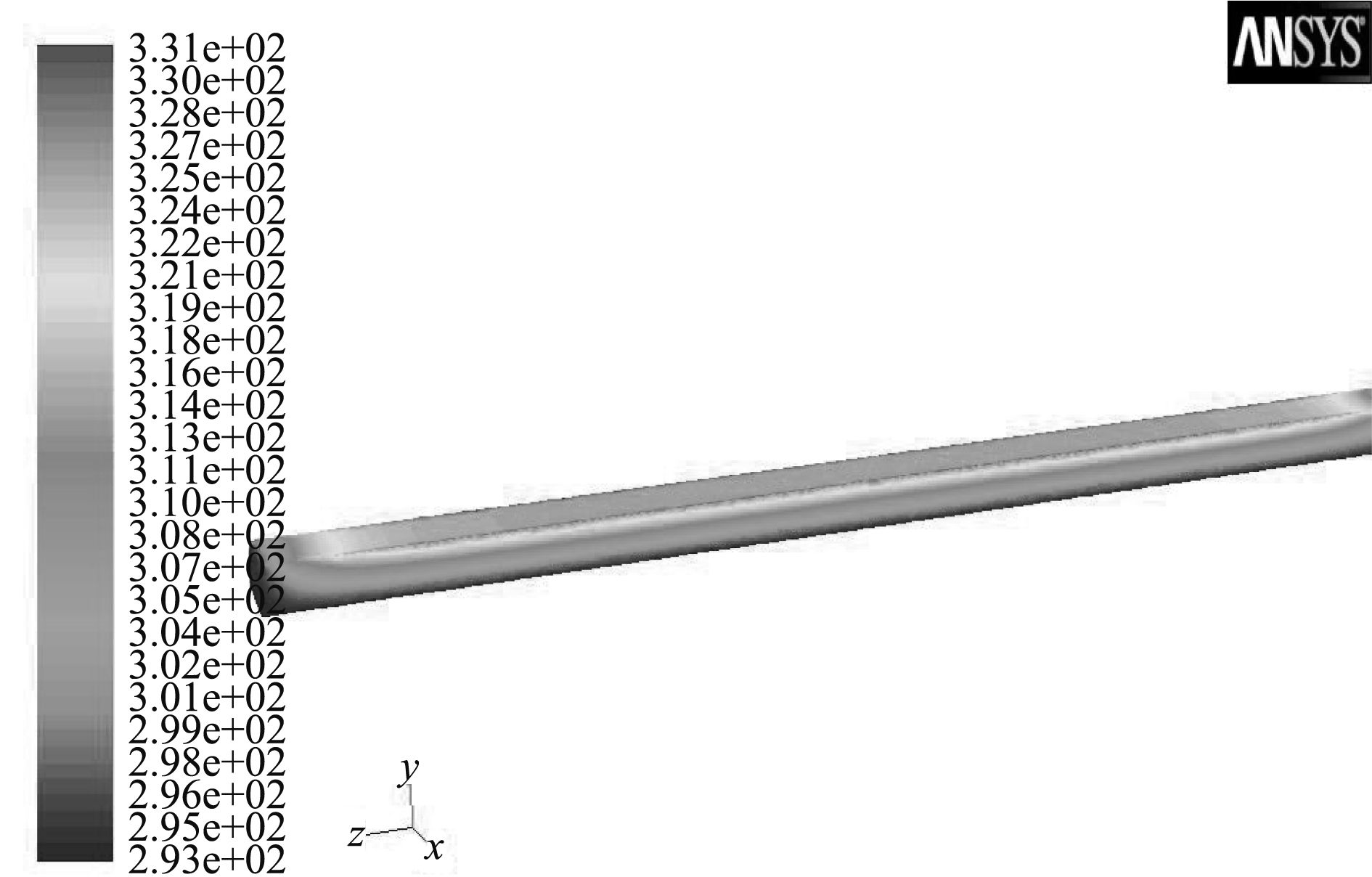图 10 外永磁体10 min温度场 Fig. 10 The 10 minutes temperature field of external peranment magnet
3.3 瞬态温度场分析

10 min时磁耦合装置最高温度出现在热源钛合金隔离罩中心处，373 K，对比初始环境温度293 K，温升为80 ℃；外磁体最高点温度58 ℃，远低于N38UH永磁体不退磁安全温度200 ℃。内永磁体温升仅为20 ℃。整个传动装置从温度云图来看，温度梯度基本呈径向变化，同一半径处轴向温度基本保持一致，装置外隔离罩圆锥部分由于浸水环境和内充空气，热阻大，导致温升不明显。支架和轴部分虽然热导率大，由于远离热源和工作时轴浸入液体的原因，温度没有显著变化。此工作时长下，钛合金隔离罩温升在合理范围内，材料强度不受影响。

4 结　语

  于雅莉, 赵波. 水下磁耦合器隔离套涡流损耗及系统传动效率分析[J]. 电工技术学报, 2014, 29 (1): 15–22.  赵克中. 磁耦合传动装置的理论与设计[M]. 北京: 化学工业出版社, 2009.  王小雷, 樊晓波. 鱼雷磁耦合传动金属隔离罩分析与优化设计[J]. 鱼雷技术, 2014, 22 (5): 347–351.  王梦文. 磁力耦合器隔离套涡流场与温度场的数值计算[J]. 机电工程, 2010 (8): 40–47.  CHARLESS J M. Magnetic Coupling Protects Machinery[J]. Design New, 1999, 7 : 26–28.  SMITH A C, WILLIAMSON S, BENHAMA A, et al. Magnetic Drive Couplings[J]. IEEE-EMD Conference, 1998, 153 (2): 289–294.  (美)英克鲁佩勒(Incropera, F. P.)等著; 葛新石, 叶宏译. 传热和传质基本原理: 第六版[M]. 北京: 化学工业出版社, 2007.